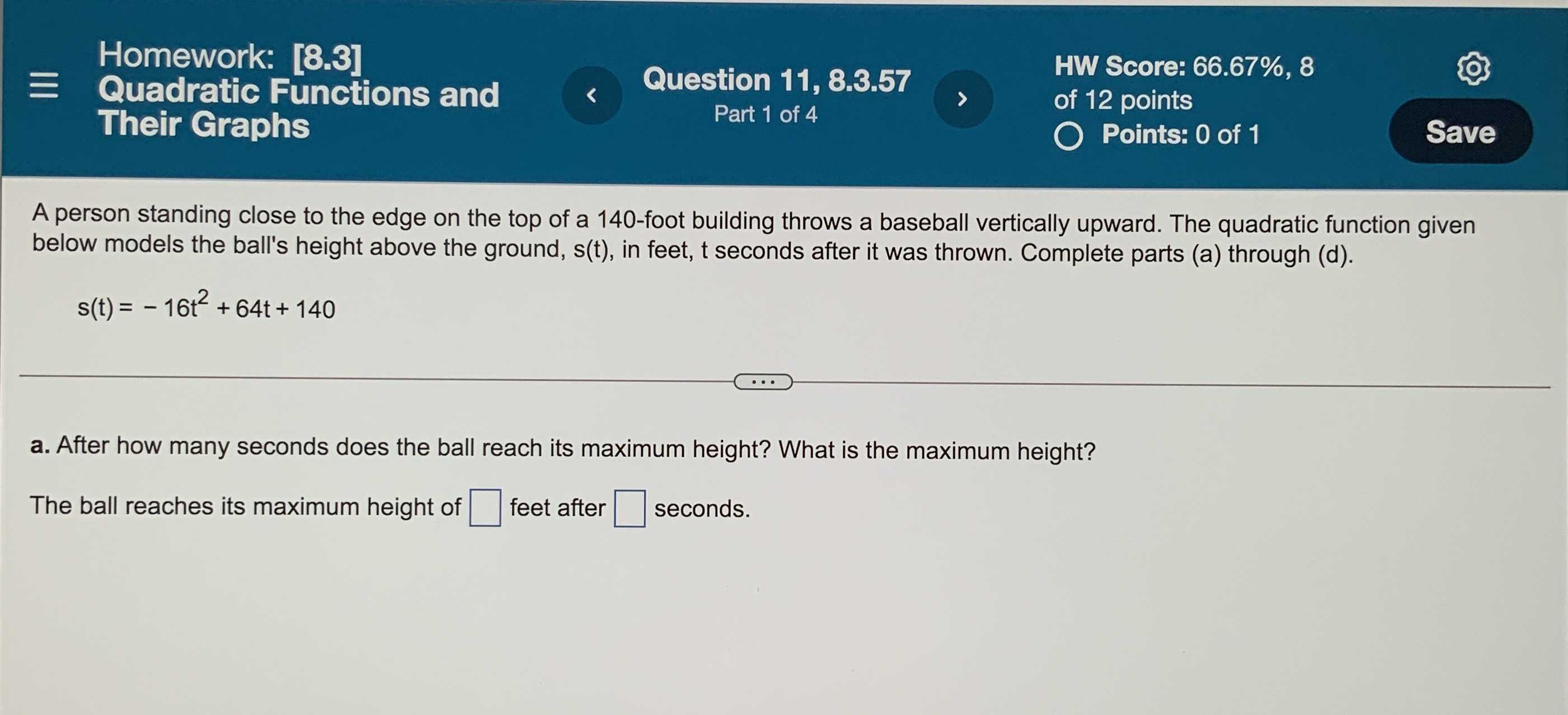### Still have math questions?

Algebra
QuestionA person standing close to the edge on the top of a $$140$$ -foot building throws a baseball vertically upward. The quadratic function given below models the ball's height above the ground, $$s ( t )$$ , in feet, $$t$$ seconds after it was thrown. Complete parts (a).

$$s ( t ) = - 16 t ^ { 2 } + 64 t + 140$$

a. After how many seconds does the ball reach its maximum height? What is the maximum height? The ball reaches its maximum height of $$\square$$ feet after $$\square$$ seconds.

$$204\ \ \ 2$$